## Cauchy DistributionThe Cauchy distribution, also called the Lorentzian Distribution, describes resonance behavior. It also describes the distribution of horizontal distances at which a Line Segment tilted at a random Angle cuts the x-Axis. Letrepresent the Angle that a line, with fixed point of rotation, makes with the vertical axis, as shown above. Then(1)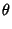(2)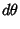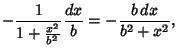(3)

so the distribution of Angleis given by(4)

This is normalized over all angles, since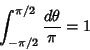(5)

and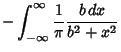(6)The general Cauchy distribution and its cumulative distribution can be written as(7)(8)

where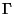is the Full Width at Half Maximum (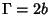in the above example) andis the Mean (in the above example). The Characteristic Function is(9)

The Moments are given by(10)(11)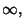(12)

and the Standard Deviation, Skewness, and Kurtosis by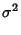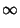(13)(14)(15)

Ifandare variates with a Normal Distribution, then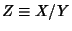has a Cauchy distribution with Meanand full width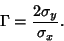(16)